6 Posts

## Subject: Dice probability problemsOk, can anyone help me out with this? I can't figure out the math behind this and really need to have a solid understanding of it for a game I am designing.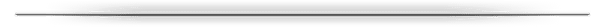What are the chances of rolling doubles on 2D6, 3D6, 4D6, etc. working your way up. For instance, if I roll 6D6, what % chance do I have of rolling a double?

What are the chances of rolling Double ones, Double sixes or double of any other numbers.

Example: If I roll 4D6, what % chance is there of rolling double 1s or double 6s (same chance), and then what is the chance of rolling double 2, 3, 4 or 5's.

The more dice you roll, the greater your chances of the doubles so I can't figure out how to get a % once I get over a few dice because I don't understand what formula to use to get the result.I tried to explain it as best I could, here is an example of how this works in the game:

Player rolls a number of D6 (that number depends on a bunch of factors, the more dice you roll the better your chance of success).

The results of the dice determine if you make a successful roll. In order for a success you need to roll a 6. ONLY a 6 is a success. A roll of a 1 is a failure. Any 1's will cancel out any 6's.

That is all the easy part, but here is where the 'math' behind it starts to give me problems. Any doubles 2's, 3's, 4's or 5's you roll become ONE success (6).

So a roll like this: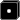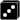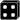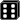Is equal to 1 failure and 2 successes (the double 3's become a success). One failure and one success cancel each other out, and you are left with one success for this roll. (The 4 is discarded since it isn't a 1 or a 6 and isn't part of a double).OVERALL QUESTION:
How do I determine my % chance of success or failure for 3d6, 4d6, 5d6, etc., etc.

Thanks!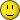• [+] Dice rolls
Tabletop Carnage wrote:
What are the chances of rolling doubles on 2D6, 3D6, 4D6, etc. working your way up. For instance, if I roll 6D6, what % chance do I have of rolling a double?
2: 1 - (5/6)
3: 1 - (5/6 * 4/6)
4: 1 - (5/6 * 4/6 * 3/6)
5: 1 - (5/6 * 4/6 * 3/6 * 2/6)
6: 1 - (5/6 * 4/6 * 3/6 * 2/6 * 1/6)

Edit:
7: Of course you have to have a doubles if you roll 7 dice (and continuing the pattern would bear that out).

But as I look at the 2nd section, I'm confused. Is that a different question? I don't see how the example relates to the 1st stated question.

My answer is for the question: What's the probability of rolling at least one doubles when rolling n dice? If you care about the value of the doubles (probability of getting a specific value of doubles), then multiply by 1/6. The probability doesn't change based on the value rolled (rolling a 1 is the same as rolling a 6 on each individual die).
• [+] Dice rolls
Here are some anydice programs!

Tabletop Carnage wrote:
What are the chances of rolling doubles on 2D6, 3D6, 4D6, etc. working your way up. For instance, if I roll 6D6, what % chance do I have of rolling a double?
https://anydice.com/program/1372c
2d6 - 16.7%
3d6 - 44.4%
4d6 - 72.2%
5d6 - 90.7%
6d6 - 98.5%

Tabletop Carnage wrote:
What are the chances of rolling Double ones, Double sixes or double of any other numbers.
Since all numbers are equally common, all doubles are, too.

https://anydice.com/program/1372d

Tabletop Carnage wrote:
I tried to explain it as best I could, here is an example of how this works in the game:

Player rolls a number of D6 (that number depends on a bunch of factors, the more dice you roll the better your chance of success).

The results of the dice determine if you make a successful roll. In order for a success you need to roll a 6. ONLY a 6 is a success. A roll of a 1 is a failure. Any 1's will cancel out any 6's.

That is all the easy part, but here is where the 'math' behind it starts to give me problems. Any doubles 2's, 3's, 4's or 5's you roll become ONE success (6).

So a roll like this:Is equal to 1 failure and 2 successes (the double 3's become a success). One failure and one success cancel each other out, and you are left with one success for this roll. (The 4 is discarded since it isn't a 1 or a 6 and isn't part of a double).
The previous answers aren't going to help you much with this, but you can still write a program for it pretty easily...

https://anydice.com/program/1372e

...I was going to write some additional commentary, but I'm short on time right now, so I'll leave it there for the moment.
• [+] Dice rolls
First, you need to be very precise in asking such questions. Your questions aren't fully defined because they mention doubles, but they don't mention triples etc.

The first part of your problem is quite easy if you mean "doubles or better". Unfortunately it won't be much help in solving the second part, your real problem. But let's look at it anyway.

I'm going to provide an example. What is the chance of a double with four dice. Or, as I'm guessing, what is the chance of a double or better with four dice.

The way to look at that is to consider a failure. How can that fail? Only if all dice are different. (It's harder if you want doubles exactly - as we then have to think about triples. Let's not do that.)

To fail, the first die may be anything. Probability 1. The next dice can only have one of five results, probability 5/6. the next dice must have one of four results, probability 4/6 and of course the last die is 3/6.

Dice are independent so we can multiply those together: 5/6 x 4/6 x 3/6 or, after cancelling out factors, 5/18.

You should be able to do other cases similarly. You could even turn it into a formula.

Now your real problem has lots of irregular detail in it. So working out a formula would be tough. It would be easier to just work through the patterns. Provided you can get a computer to do it for you.

Many people use a website called anydice. I use a program I have that's even more general purpose than anydice. Some people do things like this in Excel or write Python programs. These can all work.

But first we need an exact specification. Each 6 is a success. Each 1 is a success. Each pair of 2 to 5 is a success. Then we add up the successes, subtract the failures and get a result. If I were doing that I could produce a histogram, or any specific statistic you want.

But what if I roll three 2s? One success? What if I roll for twos? One success or two?

Complete those details and I might try running it. Won't get a formula, but an exact answer for each reasonable number of dice won't be hard with tools to hand.

But a final point - why choose this method, then have to work out the probabilities, then see if they work? Fine if you are stick with it in someone else's design, but if it's you design, why this elaborate. (Though I must admit something about it is appealing. But that was also true of something else I once analysed for someone which turned out to fail the intuitive test badly - the GM had no idea how to set difficulty levels.)
• [+] Dice rolls
Dearlove wrote:

But a final point - why choose this method, then have to work out the probabilities, then see if they work? Fine if you are stick with it in someone else's design, but if it's you design, why this elaborate. (Though I must admit something about it is appealing. But that was also true of something else I once analysed for someone which turned out to fail the intuitive test badly - the GM had no idea how to set difficulty levels.)
This is a very good point. Even if you get an answer with a formula (which I may try to work out later), I'm pretty it's going to be wonky. It's going to be hard to know how to price any adjustments to this.

Is the result of this roll binary success or failure? If so, then the only reason (that I see) you would use novel success mechanisms is because you want a particular probability shape or certain types of hooks to modify it. Otherwise, you could just use a d100 and a table. The probability properties here are going to be weird to price effects AND to know how much effects are worth in game. You don't want players breaking out a computer to run calculations.
• [+] Dice rolls
Thank you everyone for the replies. I will go over this all and get some more posted here in the next day, thanks so far!• [+] Dice rolls